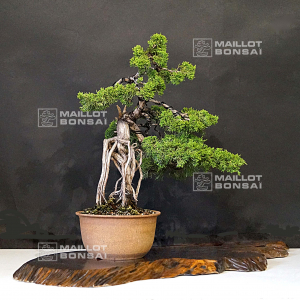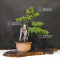##### The Japanese Bonsai specialist
Direct order Contact Help / Services Newsletter# Juniperus chinensis 25060216

› Outdoor bonsai treesref. : 10990Product unavailable

###### Description

Height of the tree only: 480 mm. Width of the branches: 400 mm.

Non-enameled pot : 200*200*110mm. Neagari style.

Coming from Master Hashimoto's nursery.

Requires generous watering in summer and regular fertilization all along the dormancy period.

This bonsaï is more than 25 years old.

It is graceful with the really natural movement of the trunk.

Without strong cutting wound nor wire mark.
Reformed in September 2019 by Master Tomoya Nishikawa : he reformed the trays to give them more proportions.

It comes from a Japanese yamadori.

Photographed in May 2021. Shelf not included.

#bonsai 3.6 #chinensis 2.9 #juniperus 2.9 #reformed 2.8 #outdoor 2.7 #master 2.6 #trees 2.5 #from 2.4 #more 2.4 #fertilization 2.3

Formule
(( ROUND((CHAR_LENGTH(b.article_nom)-CHAR_LENGTH(REPLACE(b.article_nom, 'chinensis', '')))/LENGTH('chinensis')) + ROUND((CHAR_LENGTH(b.article_description)-CHAR_LENGTH(REPLACE(b.article_description, 'chinensis', '')))/LENGTH('chinensis')) ) * 2.9) + (( ROUND((CHAR_LENGTH(b.article_nom)-CHAR_LENGTH(REPLACE(b.article_nom, 'juniperus', '')))/LENGTH('juniperus')) + ROUND((CHAR_LENGTH(b.article_description)-CHAR_LENGTH(REPLACE(b.article_description, 'juniperus', '')))/LENGTH('juniperus')) ) * 2.9) + (( ROUND((CHAR_LENGTH(b.article_nom)-CHAR_LENGTH(REPLACE(b.article_nom, 'reformed', '')))/LENGTH('reformed')) + ROUND((CHAR_LENGTH(b.article_description)-CHAR_LENGTH(REPLACE(b.article_description, 'reformed', '')))/LENGTH('reformed')) ) * 2.8) + (( ROUND((CHAR_LENGTH(b.article_nom)-CHAR_LENGTH(REPLACE(b.article_nom, 'master', '')))/LENGTH('master')) + ROUND((CHAR_LENGTH(b.article_description)-CHAR_LENGTH(REPLACE(b.article_description, 'master', '')))/LENGTH('master')) ) * 2.6) + (( ROUND((CHAR_LENGTH(b.article_nom)-CHAR_LENGTH(REPLACE(b.article_nom, 'more', '')))/LENGTH('more')) + ROUND((CHAR_LENGTH(b.article_description)-CHAR_LENGTH(REPLACE(b.article_description, 'more', '')))/LENGTH('more')) ) * 2.4) + (( ROUND((CHAR_LENGTH(b.article_nom)-CHAR_LENGTH(REPLACE(b.article_nom, 'from', '')))/LENGTH('from')) + ROUND((CHAR_LENGTH(b.article_description)-CHAR_LENGTH(REPLACE(b.article_description, 'from', '')))/LENGTH('from')) ) * 2.4) + (( ROUND((CHAR_LENGTH(b.article_nom)-CHAR_LENGTH(REPLACE(b.article_nom, 'fertilization', '')))/LENGTH('fertilization')) + ROUND((CHAR_LENGTH(b.article_description)-CHAR_LENGTH(REPLACE(b.article_description, 'fertilization', '')))/LENGTH('fertilization')) ) * 2.3) + (( ROUND((CHAR_LENGTH(b.article_nom)-CHAR_LENGTH(REPLACE(b.article_nom, 'photographed', '')))/LENGTH('photographed')) + ROUND((CHAR_LENGTH(b.article_description)-CHAR_LENGTH(REPLACE(b.article_description, 'photographed', '')))/LENGTH('photographed')) ) * 2.2) + (( ROUND((CHAR_LENGTH(b.article_nom)-CHAR_LENGTH(REPLACE(b.article_nom, 'proportions', '')))/LENGTH('proportions')) + ROUND((CHAR_LENGTH(b.article_description)-CHAR_LENGTH(REPLACE(b.article_description, 'proportions', '')))/LENGTH('proportions')) ) * 2.1) + (( ROUND((CHAR_LENGTH(b.article_nom)-CHAR_LENGTH(REPLACE(b.article_nom, 'september', '')))/LENGTH('september')) + ROUND((CHAR_LENGTH(b.article_description)-CHAR_LENGTH(REPLACE(b.article_description, 'september', '')))/LENGTH('september')) ) * 1.9)

## Secure payment## Delivery

Our logistic partners :04 74 55 23 48
Pépinière MAILLOT-BONSAÏ
Le Bois Frazy
01990 RELEVANT - FRANCE
on appointment